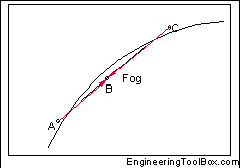# Mixing air how much water leaving?

peet_dk
I have a situation like this:Where C is a air stream from a pipe, and A is the sky. Droplet will be made, and there will be fog.

Now I have to calculate the amount of water leaving the air.

In my book I found this equation:

Qwater = Qdry.air.C * (xA - xC)

But do it have to be point C and A? Why not the two points where RH=100% ?

And how do I know how much water that there will be in the air as fog, and how much will raining down?

## Answers and Replies

peet_dk
I made a pdf of the diagram, with more details on it.

#### Attachments

• hx-Diagramm.pdf
24.1 KB · Views: 321